一道数组面试题-不能使用辅助空间找重复次数的数

年薪达不到25W大数据工程师、拿不到Offer全额退款->>>ps:提示各位面试的时候一定要复习基础知识。不然若如果你在某个领域上做了好几年。肯定忘记了一些基础知识，但是面试的时候就有可能遇到。比如 java的锁。当年读大学的时候我还很深入的研究过操作系统的各种锁机制和java的锁机制。毕业几年了。写j2ee很少遇到自己写锁同步的事情。结果都荒废了。面试的时候问到了，都几乎搭不上话。丢脸

0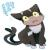0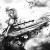import java.util.Random;

public class FindMax {
int[] source = new int;

public FindMax() {
Random rand = new Random();
for (int i = 0; i < 50; i++) {
source[i] = rand.nextInt(20) + 1;
}
}

public int getMaxTest() {
int[] check = new int;
for (int i = 0; i < 20; i++) {
check[i] = 0;
}
int max = 0;
for (int i = 0; i < 50; i++) {
check[source[i] - 1] += 1;
if (check[max] < check[source[i] - 1]) {
max = source[i] - 1;
}
}
return max + 1;
}

public int getMax() {
for (; getExtra(21) < 50; setExtra(21, getExtra(21) + 1)) {
setExtra(getBase(getExtra(21)), getExtra(getBase(getExtra(21))) + 1);
if (getExtra(getExtra(0)) < getExtra(getBase(getExtra(21)))) {
setExtra(0, getBase(getExtra(21)));
}
}
return getExtra(0);
}

public int getBase(int i) {
return source[i] % 100;
}

public int getExtra(int i) {
return source[i] / 100;
}

public void setExtra(int i, int extra) {
source[i] = extra * 100 + getBase(i);
}

public static void main(String[] args) {
FindMax fm = new FindMax();
System.out.println(fm.getMaxTest());
System.out.println(fm.getMax());
}
}

0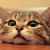0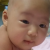0for (i=0; i<50; i++)

{

x=array[i];

n=array[x]>>4;

array[x] |= (n+1)<<4;

};

max=0;

for (i=0; i<20; i++)

if (  (array[i]>>4  ) >( array[max]>>4)

max=i;

printf( max);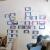0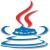public class sb {

public static void findTheShit(int[] array) {
for(int i = 0; i < array.length; i++)
array[array[i] % 20 ==0 ? 20 : (array[i] % 20)] += 20;

array = 0;
for(int i = 1; i <= 20; i++) {
if(array < (int)((array[i] - 1) / 20))
array = (int)((array[i] - 1) / 20);
}

System.out.println("---出现次数最多的数字(" + array  + "次)---");
for(int i = 1; i <= 20; i++) {
if(array * 20 < array[i])
System.out.println(i);
}
}

public static void main(String[] args) {
int[] rand = new int;
for(int i = 0; i < 50; i++) {
rand[i] = (int)(Math.random() * 20) + 1;
System.out.print(rand[i] + "\t");
}
System.out.println();
findTheShit(rand);
}
}

0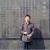引用来自“卖切糕大叔”的答案

public class sb {

public static void findTheShit(int[] array) {
for(int i = 0; i < array.length; i++)
array[array[i] % 20 ==0 ? 20 : (array[i] % 20)] += 20;

array = 0;
for(int i = 1; i <= 20; i++) {
if(array < (int)((array[i] - 1) / 20))
array = (int)((array[i] - 1) / 20);
}

System.out.println("---出现次数最多的数字(" + array  + "次)---");
for(int i = 1; i <= 20; i++) {
if(array * 20 < array[i])
System.out.println(i);
}
}

public static void main(String[] args) {
int[] rand = new int;
for(int i = 0; i < 50; i++) {
rand[i] = (int)(Math.random() * 20) + 1;
System.out.print(rand[i] + "\t");
}
System.out.println();
findTheShit(rand);
}
}

void calc_max_num(int *a, int n){
int i = 0;

while(i < n){
a[a[i] % 20] += 20;
++i;
}

a[n-1] = 0;//存放出现的次数最大值
a[n-2] = 0;//存放出现次数最多的数值

i = 0;
while( i < 20){
if(a[i]/20 > a[n-1]){
a[n-2] = i;
a[n-1] = a[i]/20;

}
++i;
}
printf("The num is: %d\n", a[n-2] == 0 ? 20 : a[n-2]);
printf("The max occurrence is: %d\n", a[n-1]);

}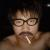0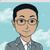0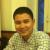0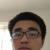#include <iostream>
#include <ctime>
#include <cstdlib>
using namespace std;

int main()
{
//Generate 50 numbers ranging from 1-20
srand( clock() );

int a;
int counter = {0};

cout<<"Original array:"<<endl;
for( int i=0;i<50;i++ ) {
a[i] = rand()%20+1;
cout<<a[i]<<" ";
counter[a[i]-1]++;
}
cout<<"\n===== END ======"<<endl;

// Dumb method to check real top number
{
int maxCounter = 0;
int maxNumber = 0;
for( int i=0;i<20; i++ )
{
if( counter[i]>maxCounter ) {
maxCounter = counter[i];
maxNumber = i+1;
}
}
cout<<" Real max number:"<<maxNumber<<" appear="<<maxCounter<<endl;
}

// Raise previous 20 elements by 6 bit as counter, as 2^64>50
for( int i=0;i<20;i++ ) a[i]<<=6;

// Count previous 20 elements
for( int i=0;i<20;i++ ) a[ ((a[i]>>6)-1) ]++;

for( int i=20;i<50;i++ ) a[ a[i]-1 ] ++;

// Take a as max counter
int &maxCnt = a;
// Remember max in a
int &maxNumber = a;

maxCnt = 0;
maxNumber = 0;
for( int i=0;i<20;i++ ) {
if( (a[i]&0x3f) >maxCnt )
{
maxCnt = a[i]&0x3f;
maxNumber = i+1;
}
}

cout<<"The max number is: "<<maxNumber<<" appear="<<maxCnt<<endl;
return 0;
}